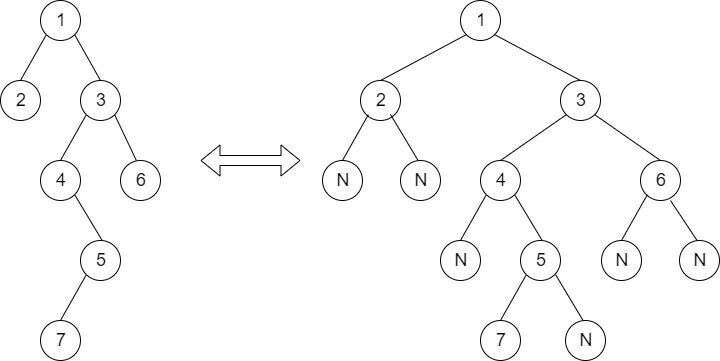Check if two Nodes are Cousins
##### Submissions: 9803   Accuracy: 37.33%   Difficulty: Easy   Marks: 2

Given the binary Tree of size N and the two-node value say ‘a’ and ‘b’, determine whether the two-node values are cousins of each other or not. The values of all the nodes in the tree are distinct.

Two nodes value are cousins of each other if they are at the same level and have different parents.

Input:
The first line of input contains the number of test cases T. For each test case, there will be two lines of input, First input is a string representing the tree as described below and the second line contains two space-separated integers denoting node values:

1. The values in the string are in the order of level order traversal of the tree where, numbers denote node values, and a character “N” denotes NULL child.

2. For example:For the above tree, the string will be: 1 2 3 N N 4 6 N 5 N N 7 N

Output:
Single line output, print "1" if they are cousins else "0".
You don't need to take input. Just complete the function isCousins() that accepts the root node of the tree, and two integers as a parameter and returns true, if two integers are cousins else returns false.

Constraints:
1<=T<=1000
1<=N<=1000

Example:
Input:

2
1 2 3
2 3
1 2 3 5 N N 4
4 5
Output:
0
1

Note: The Input/Ouput format and Example given are used for system's internal purpose, and should be used by a user for Expected Output only. As it is a function problem, hence a user should not read any input from stdin/console. The task is to complete the function specified, and not to write the full code.

#### ** For More Input/Output Examples Use 'Expected Output' option **

Contributor: Saksham Raj Seth
Author: saksham seth

If you have purchased any course from GeeksforGeeks then please ask your doubt on course discussion forum. You will get quick replies from GFG Moderators there.